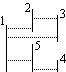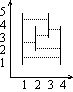시간 제한 메모리 제한 제출 정답 맞은 사람 정답 비율
1 초 128 MB 0 0 0 0.000%

## 문제

Lets consider a set S of n vertical segments on the plain (segments include their end points). None of the elements of S have a point in common. Two segments are said to "see each other" if there exists a horizontal segment, which connects them and does not have any common points with other segments from S.

On the figure below, there is an example set of segments. Pairs of segments that can see each other, are: 1-2, 1-3, 2-3, 1-5 and 4-5. The segments 1 and 4 do not see each other.For a given number n, we search for a set S of n vertical segments such that the number of pairs of segments which can see each other is as big as possible.

Write a program which:

• reads the number n from the standard input,
• computes locations of n vertical segments, such that
• none of them have a point in common,
• the number of pairs of segments which can see each other is as big as possible,
• writes the answer to the standard output.

## 입력

The first and only line of the standard input contains one integer n (1 ≤ n ≤ 20 000).

## 출력

The first line of the standard output should contain one integer - maximal number of pairs of segments that can see each other. Each of the following n lines should identify the coordinates of one segment. Line i + 1 (for i = 1, 2, ..., n) consists of three integers: xi, di and gi (0 ≤ xi ≤ 1 000 000 000, 0 ≤ di < gi ≤ 1 000 000 000), separated by a single space. They represent the segment which connects the points (xi, di) and (xi, gi).

If there are more than one correct arrangement of n segments, your program can write out any of them.

## 예제 입력 1

4


## 예제 출력 1

6
1 1 5
2 2 4
3 3 5
4 1 4


## 힌트